# A neural tool for the prediction of the experimental dynamics of two-phase flows

A neural tool for the prediction of the experimental dynamics of two-phase flows

Alberto Fichera Arturo Pagano

DIEEI, Università degli Studi di Catania, viale A. Doria 6, 95125 Catania, Italy

Corresponding Author Email:
arturo.pagano@dieei.unict.it
Page:
235-242
|
DOI:
https://doi.org/10.18280/ijht.350201
|
Accepted:
|
Published:
30 June 2017
| Citation

OPEN ACCESS

Abstract:

The dynamics exhibited by two-phase flows, which manifest themselves in a great variety of different flow patterns, are intrinsically complex due to the relevant number of degree of freedom, the nonlinear interactionof several phenomena and the uncertainty on the physical parameters. Therefore, an exhaustive mathematical modelling of two-phase flow dynamics is very difficult not only to assess and validate but also to extend and generalize to other applications. Nonetheless, a reliable model specifically oriented to the prediction of such dynamics, would represent an interesting step ahead towards the possibility of developing diagnostic or control tools for a variety of two-phase flow applications. The present study proposes the assessment of a short term prediction model derived from the experimental time series of the void fraction detected during an extensive experimental campaign for the characterization of vertical upward air-water two-phase flows under variable water and air superficial velocities, wsl and wsg. The identification strategy relies on the assessment of a NARMAX model (Nonlinear AutoRegressive Moving Average with eXogenous inputs) implemented in an approximated and generalized form by means of an optimized Multilayer Perceptron artificial neural network. Reported results show that a satisfactory agreement is reached between simulated and experimental data, showing that the model successfully predicts the time evolution of the void fraction dynamics. The application of a recursive feedback scheme to the model outputs allows to observe that satisfactory predictions can be obtained also for multiple steps ahead, though in the limits of a short-term predictability.

Keywords:

dynamical model, neural identification, short-term prediction, two-phase flow

1. Introduction

The occurrence of two-phase flows in pipes, is a characterizing feature of a variety of physical phenomena as well as of several fundamental components of industrial plants with applications in power generation, oil, chemical and process plants, food industry and many others. Even restricting to the case of non-reacting phases and in the absence of phase changes, the nature of this kind of flows is extremely complex. This is due, in fact, to the coexistence and the nonlinear interaction of several physical effects, such as buoyancy, turbulence, inertia, surface-tension forces, which, in turn, affect the mechanisms of pattern formation as well as those governing instability generation and propagation.

As a consequence, it is very difficult to describe with great accuracy two-phase flows in specific systems. Moreover, even when such descriptions are obtained, it is not easy to generalize their validity to account for other systems, due to the fundamental role played by the thermo-physical properties of the two phases, by geometrical parameters, such as the pipe inclination, the cross-section and the hydraulic diameter, but also by the nature of the observed variables and of the measurement technique correspondingly adopted.

This justifies the great effort towards the possibility of realizing “black box” identification tools, often based on a variety of strategies assessed within the field of artificial intelligence, as summarized in . In fact, on the basis of limited information on the regime of a given system, the identification approach is often able to accurately predict some fundamental characteristics of the two-phase process, such as the holdup, the pressure drop or the type of flow pattern. Several study have shown how artificial neural networks, ANNs, are particularly appropriate for this purpose due to their simple implementation and generalization capability.

In fact, referring to the class of two-phase flow in the absence of phase changes, neural models have been proposed for the prediction of fluid-dynamical outputs, such as pressure drop, liquid holdup, pressure gradient or void fraction correlation [2-9], but also for heat transfer coefficients or flow pattern recognition [10-12]. Great interest towards neural tools has been shown also in the field of nuclear reactors, leading to several neural models for the identification not only of the outputs previously mentioned but, more specifically, of characteristic thermal aspects of boiling phenomena, such as the onset of nucleate boiling, the critical heat flux and the boiling curve, extensively reviewed in .

For all of the reported cases the predicted outputs represent global characteristics of the flow, i.e. they are synthetic expression of time-averaged flow characteristics, which do indirectly depend on the dynamics but are not able to describe the evolution in time. As a matter of fact, due to their inherent complexity, the prediction of the time evolution of two-phase flows is a very onerous task that has been addressed on the basis of numerical models. Nonetheless, these are either computationally intensive, as it happens for distributed (i.e. PDE) models, or affected by relevant simplifications, as it happens for models which describe two-phase flows by means of low order ODEs . As a consequence, such models are not always suitable for direct application as diagnostic tools for industrial system and there is interest towards the possibility of designing flexible, accurate and computationally light tools for the prediction of two-phase flow dynamics.

Therefore, the aim of the present study is to extend the use of neural models, trained to learn from experimental examples, in order to realize a tool for the short-term prediction of the dynamics of two-phase flow. From a mathematical standpoint, the general framework for the assessment of identification models for nonlinear dynamical systems is represented by the NARMAX strategy (Nonlinear Auto-Regressive Moving Average with eXogenous input) [15-16]. This approach would require a specific model for each of the possible dynamics of a given system; nonetheless, a single model valid in the entire range of the dynamics expressed by the system, namely a generalized NARMAX model, can be efficiently and flexibly implemented by a Multilayer Perceptron neural network.

The training and testing of the neural model has been based on the experimental time series of the void fraction detected by a high resolution resistive probe during an extensive campaign for the characterization of the dynamics of air- water two-phase flows ascending in a vertical circular pipe, as reported in . Some preliminary results of the proposed strategy were reported on . The aim of the present study is to propose a detailed discussion on the application of the identification strategy and to extend and fully report the obtained results.

2. Narmax Identification

The NARMAX (Nonlinear Auto-Regressive Moving Average with eXogenous inputs) identification represents a general strategy for the assessment of non-linear input-output models [15-16] of discrete time invariant non-linear systems that are characterized by having lumped parameters and that can be linearized in a neighborhood of their equilibria . The main advantage of the strategy consists in the possibility to realize a predictive tool just on the basis of experimental time series, i.e. of input-output measurements, even without any initial knowledge of the physics of the observed nonlinear system. In particular, the ability in the prediction of the time evolution of one or more of the system outputs, makes this instrument very interesting for a variety of monitoring, diagnostic and control tasks, especially when parameter variations and uncertainty are strong and drastically affect the reliability of other modeling approaches based on an explicit mathematical description of the physics of the system.

In fact, the identification consists in a parametric assessment of a non-linear function, F, which predicts a system output y(k) at a given time sample k, from available information on lagged inputs and outputs of the system, measured at previous time steps. In accordance to the detailed descriptions reported in [20-21], the NARMAX identification of the output y(k) at time k of a SISO (Single Input - Single Output) system, can be expressed as:

y(k)=F[y(k-1), …, y(k-ny), u(k-1), …, u(k-nu)]      (1)

where u(▪) and y(▪) at the right-hand side are lagged inputs and outputs, respectively, at previous time samples, with nu depending on the nature of the input modulation and ny representing the model order. This function is a valid representation of the system just for the dynamical states belonging to a neighborhood of the specific equilibrium on which the linearized model is defined. Nonetheless, this limit is very efficiently overcome thanks to the interpolation ability of neural networks. In fact, a generalized NARMAX model,

i.e. a model valid for a variety of different equilibria and even for piece-wise linear function F, can be approximated by means of a Multi-Layer Perceptron Neural Network. Moreover, such a choice is advantageous also because the nonlinear input-output relation of a neural network allows to bypass the linearization around the system equilibria.

With respect to classical modelling approaches, based on mathematical descriptions, the parametric assessment of the neural model is extremely simple and consists in learning by known examples of the system dynamics during a training phase. In particular, during the training of the neural model, some input vectors are fed to the network and the calculated outputs are compared with the desired targets corresponding to the inputs; afterwards, the weights of the neural network, which are the unknown parameters of the model and are randomly assigned at the beginning of the training, are recursively updated according to a specified training algorithm that aims at minimising a cost function, usually corresponding or related to the prediction error. In a general mathematical framework, if a network with n inputs and m outputs is considered, its global input-output relationship is a function NN:Rn ® Rm. Cybenko  and Funahashi  demonstrated that, at the end of an appropriate training the neural model NN:Rn ® Rm is able to uniformly approximate any continuous function F:D Ì Rn ® Rm, where D is a compact subset of Rn, so that equation (1) can be rewritten as:

y(k) » NN[y(k-1), …, y(k-ny), u(k-1), …, u(k-nu)]      (2)

In other terms, if the training examples are sufficiently representative of the set of possible dynamical conditions, once that the training of the neural network has been completed a generalised NARMAX neural model is obtained that should be able to simulate the entire set of the system dynamics encompassed by the conditions learned during the training. Therefore, the prediction capability of the neural model is typically verified on a testing data set not overlapping with the training data set. In other terms, the testing data set is formed by a series of input patterns and of the corresponding output targets, similarly to the training set, but is built with pieces of information corresponding to dynamics unknown to the neural network.

The performances of the model can be analytically assessed through the characterization of the prediction error,defined as the difference between experimental and simulated time series. In general, the prediction of a model is satisfactory when the normalized error e(k) has zero mean and is uncorrelated . Such a condition can be usually assumed to be fulfilled when the autocorrelation function of the normalised error, fee(k), assumes fee(0)=1 at time k=0 and is elsewhere confined within a 95% confidence band centred on fee(k)=0, which, calculating fee(t) over a window of N samples, corresponds to the range ± 1.96 N-0.5.

3. Application of the Narmax Approach to the Prediction of Void Fraction Dynamics

In this study, the generalised NARMAX approach is used in order to predict the experimental dynamics of air-water two-phase flows in upward motion in vertical pipes. Full details are reported in  concerning the experimental apparatus and on the extensive set of experimental testing conditions carried out by varying the air and water superficial velocities within the entire range of their possible values. The observed variable chosen for the analysis and characterisation of the flow dynamics is the void fraction, which expresses the volume fraction of air with respect to the total volume occupied by the two phases. This was measured by means of a resistive probe positioned at a distance of over 100 times the diameter of the pipe from the mixing section in order to ensure the observation of well-established flow regimes, i.e. over the required entry region for two phase flows. In order to ensure a high spatial and temporal resolution, the void fraction probe was specifically designed and realised for the experimental campaign. It consists of an impedance probe operating in the resistive range. The sampling frequency was set at 1 kHz and a cut-off frequency of 200 Hz was adopted in order to allow the removal of the carrier frequency and to avoid aliasing.

With respect to the observed variable, the general structure of the NARMAX model expressed by equation (1) can be reformulated for the case of interest as:

[vf(k)] = F [vf(k-1), vf(k-2), … , vf(k-ny), wsl, wsg] (3) where vf(k) represents the measured void fraction at time k,

i.e. the output of the system that the model aims to predict, whereas the inputs wsl and wsg represent the superficial velocities of the liquid and gas phases, respectively. It is worth observing that, in the present study, wsl and wsg are not time dependent inputs for a given dynamical pattern, as both the training and testing data set were extracted by experiments performed at constant values of these terms.

Previous analyses of the experimental time series pointed out that the dominant features of two-phase flow dynamics are appropriately characterized by a limited number of eigenvectors ; this implies that a low order is expected for the NARMAX model, which corresponds to a limited number of lagged outputs, ny, that is necessary to set in equation (3) in order to obtain sufficiently accurate predictions. Nonetheless, though this value is unknown, its choice can be performed on the basis of a heuristic and more reliable strategy, as proposed in . In fact, the order of the model can be progressively increased, starting from ny=1, until a beneficial effect is observed on the autocorrelation of the prediction error. In fact, the most the error is uncorrelated, the best is the prediction performance of the neural network, as a low correlation implies that the model is able to capture the

predictable part of the system dynamics. As soon as the growth of ny is not effective, or even detrimental, its previous value can be set as the appropriate model order. Parenthetically, allowing the determination of the unknown order of the model, the proposed approach can be adopted as an initial step for the assessment of other types of model where such an information is preliminary required.

For each model order, a family of three-layered neural networks was trained, differing for the number of neurons constituting the hidden layer. The input layer is made up of a number of neurons corresponding to the arguments of F( . ) on the right-hand side of equation (3). These input neurons do not perform any transformation of their input values, which are transferred through weighted connections to all of the neurons of the hidden layer. These implement the tangent- sigmoid function and, hence, perform a nonlinear transformation of their inputs, each of which is obtained as the sum of the outputs of the previous layer weighted through their connections. The third layer of the network, by its turn, is made up of a single neuron that implements a linear transformation of the weighted sum of the outputs of the hidden layer and gives in output the model prediction, i.e. the void fraction, vf, at the left-hand side of equation (3). As for the choice of the best order, the optimal neural model within a family of neural networks of the same order, was found by increasing the number of hidden neurons until this is beneficial with respect to the prediction error.

Table 1. Experimental conditions encompassed in the training and testing data sets.

 Experimental condition Training Set Testing Set wsl [m/s] wsg [m/s] wsl [m/s] wsg [m/s] 1 0.002 0.031 0.345 0.031 2 0.588 0.031 0.769 0.031 3 1.550 0.031 1.202 0.031 4 0.273 0.063 0.559 0.063 5 1.085 0.063 0.834 0.063 6 0.603 0.094 0.866 0.094 7 0.117 0.126 0.499 0.126 8 1.092 0.126 0.684 0.157 9 0.002 0.157 0.121 0.188 10 0.925 0.157 0.757 0.188 11 0.215 0.220 0.509 0.220 12 1.038 0.220 0.247 0.251 13 0.090 0.283 1.038 0.283 14 1.290 0.283 0.772 0.314 15 0.032 0.314 1.123 0.471 16 1.217 0.314 0.484 0.628 17 0.075 0.942 1.064 0.628 18 1.592 0.942 0.693 0.942 19 0.060 1.570 0.205 1.256 20 0.693 1.570 0.482 1.256 21 1.516 1.570 0.940 1.256 22 0.075 2.511 0.463 1.884 23 0.452 2.511 0.891 1.884 24 1.488 2.511 0.134 2.197 25 0.122 2.825 0.678 2.197 26 0.753 2.825 1.230 2.197 27 1.424 2.825 0.878 2.511

For each model order, ny, the training set is constituted of a matrix having in its rows the training patterns and a target vector, made up of the desired target corresponding to each pattern. The patterns were selected as pieces of the void fraction time series encompassing ny time steps, while the corresponding target was the one-step ahead value of the time series. The pattern-target couples used to train the network were selected from the 27 experimental conditions sparsely but thoroughly distributed over the entire set of 476 observed conditions reported in , with the aim of encompassing a sufficiently representative sample of the dynamics observed at different values of the mean superficial velocity of the liquid phase, wsl, and of the gas phase, wsg. For each of the mentioned 27 experimental conditions, three separated windows of 1000 samples were selected by the void fraction time series. In particular, these windows were chosen with the aim of describing different but highly recurrent waveforms within the time series. Moreover, in order to reduce the dimension of the training data set, each piece of time series was preliminary decimated considering 1 out of 3 data, for an actual length of 333 data; this means that the training goal is focused on the dominant patterns of the dynamics while high- frequency noisy components are cut-off. Such a choice corresponds to setting the model time step, k, equal to 3 ms.

In order to validate the predictive performances of the NARMAX model, a testing data set was created on the basis of the same criteria and with the same dimension chosen for the training set, but extending the pattern-target couples selection to the entire set of experimental conditions, in order to verify the generalization capability of the NARMAX model in the prediction of dynamics observed for values of wsl and wsg different from those learned during the training.

The neural models were trained feeding it in batching, in order to ensure higher training performances, and using the Levenberg–Marquardt training algorithm . The number of epochs varied from network to network because the training was performed with a contemporary testing of the network prediction with a checking data set, analogous to the testing data set and, hence, not participating to the adjustment of the neural network weights. This allows to validate the training and to avoid over-fitting, i.e. the tendency of a neural model to forcibly reproduce the learned dynamical behaviors, therefore contrasting to desired generalization attitude. On the basis of this choice, the training phase is automatically ended not through the choice of a predefined number of epochs or as soon as a desired minimum of the error is reached but when the testing performances on unlearned patterns get worse at one epoch with respect to the previous.

4. Results and Discussion

As discussed in the previous section, a family of network was obtained for each model order by varying the number of hidden neurons, so as to allow the choice of the optimal neural network on the basis of error analysis.

As an example, Table 2 reports the maximum absolute value of the prediction error for the family of networks implementing the sixth order model, as well as the results of the analysis of the error autocorrelation fee(k). As previously discussed, the error was considered uncorrelated when fee(k) assumed fee(k)=1 at time k=0 and was elsewhere confined within a 95% confidence band centred on fee(k)=0. Hence, it appears from reported results that the network with 11 hidden neurons can be choose as the best performing for the sixth

order model. In a similar fashion, the optimal model order can be obtained by increasing its value until this corresponds to an improvement of the prediction performances, i.e. to a reduction of the model error.

Table 2. Prediction errors for neural model of order ny=6.In grey, the network with optimal performances

 Hidden neurons Max(|error|) Error autocorrelation Model order 6 8 0.1945 Correlated error 9 0.1832 Uncorrelated error 10 0.1874 Uncorrelated error 11 0.1687 Uncorrelated error 12 0.1889 Uncorrelated error 13 0.2032 Correlated error 14 0.2163 Correlated error 15 0.2714 Correlated error

Table 3. Prediction error for the optimal neural networks for increasing order. In grey, the optimal model, for ny=6.

 Model order Hidden neurons (optimal) Max (|error|) Error autocorrelation 4 12 0.1794 Correlated error 5 11 0.1811 Correlated error 6 11 0.1687 Uncorrelated error 7 12 0.1889 Uncorrelated error 8 12 0.2163 Uncorrelated error 9 20 0.2714 Correlated error

Table 3 reports the synthesis of the best neural networks at the various model orders and allows to state that the sixth order neural network with 11 hidden neurons is the one that best approximate the generalized NARMAX model defined in equation (3). Therefore, the results reported in the following refer to this optimal model.

Figure 1 shows the comparison between the experimental time series of void fraction and the corresponding neural network prediction in the experimental condition 21 of the testing set (columns on the right-hand side of Table 1, corresponding to a slug flow observed during the experiment conducted with superficial liquid velocity wsl=0.940 m/s and superficial gas velocity wsg=1.256 m/s. With respect to the void fraction time series reported in Figure 1, it is useful to point out that its structure is clearly related to the transit of air bubbles alternated to liquid slugs. In particular, the passage of the head of an air bubble is related to the initial progressive growth of the measured void fraction, which reaches a value that depends on the thickness of the liquid film enveloping the bubble itself.

Afterwards, along the central region of the bubble, the measured void fraction is relatively uniform, though relevant oscillations may occur, especially if this region is sufficiently developed, due both to waves travelling at the interface between the air and its liquid envelope and to small air bubbles aerating the liquid film. Finally, at the passage of the tail region, the measured void fraction abruptly decreases to approximately zero, though perturbations may occur due to the passage of small dispersed bubbles entrained in the liquid slug that follows the bubble.

The second plot of Figure 1 reports the prediction error calculated as the difference between the testing target and the output predicted by the optimal generalized NARMAX model for testing condition 21, corresponding to a slug flow

observed at superficial liquid velocity wsl=0.940 m/s and gas velocity wsg=1.256 m/s. The third plot reports the autocorrelation function of the prediction error, fee(k). Results reported in Figure 1 points out that the neural network predictions are indeed satisfactory and indicate that the training was appropriate and satisfactory ensured generalization capabilities. In particular, even if the observed experimental time series are complex, as manifested by their non-periodic nature, the prediction error is nonetheless sufficiently low and uncorrelated, demonstrating that the neural model is able to capture the deterministic components of the observed dynamics.

## 1.png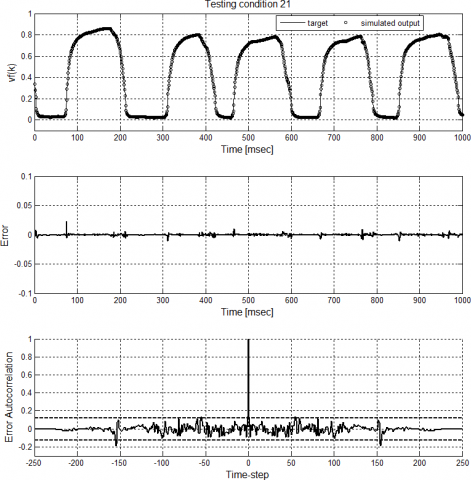Figure 1 Model results for testing condition 21 (from top): experimental target vs simulated output; prediction error;error autocorrelation.

This observation is reinforced by the analogous comparisons reported in Figure 2 for the experimental conditions 6 and 16 of the testing data set, respectively corresponding to a cap flow and to a plug flow.

It is just mentioned that, though not reported, the analysis of the error autocorrelation showed also for these conditions that the prediction error is uncorrelated. Instead, what is worth observing here is that this result is not surprising if one accounts that the slug flow reported in Figure 1 and the cap and the plug flows reported in Figure 2 can be considered to share an analogous degree of complexity. In particular, it is apparent that their characteristic oscillations have similar morphology; in fact, they are determined by a non-periodic yet relatively order alternate pulsating flow of air bubbles and liquid slugs, being the main differences among them related to the length and the diameter of the air bubble.

On the other hand, the comparisons between the experimental target and the model prediction in Figure 3 refer to more complex time series that characterize the experimental dynamics, respectively, of test number 24 of the testing data set, corresponding to a churn flow, and of test 27, corresponding to an unstable slug flow.

Parenthetically, in the churn flow and in the unstable slug flow the liquid film may continue to drain down the wall, as in the plug flow, and occasionally the travelling waves at the interface between the two phases may bridge with each other,causing the liquid envelope to collapse within the tube. Nonetheless, considering the high turbulence of this flow regime, the liquid phase is typically highly aerated and, hence, the void fraction is never too close to zero. Again, also for these cases the error was observed to be uncorrelated and, hence, notwithstanding the greater complexity, also for such kinds of flow patterns the sixth order NARMAX model is able to satisfactorily predict the flow dynamics.

More in general, a common feature of the results reported insofar is that, though the prediction error is generally very low, it is occasionally affected by “trains” or “pockets” of perturbations characterized by relatively higher amplitudes compared to those of the dominant patterns of the void fraction time series. At a deeper insight it is possible to observe that these perturbations are due to the gas phase dispersed in small bubbles entrapped in the liquid phase.

## 2.1.png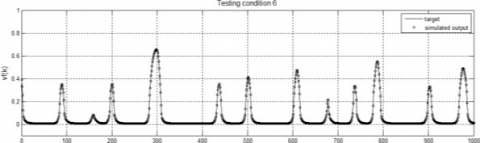## 2.2.png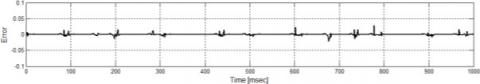## 2.3.png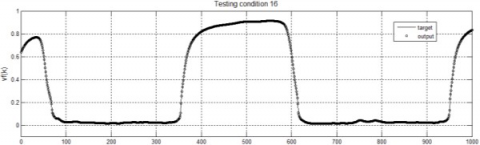## 2.4.pngFigure 2. Target vs simulated output and prediction error for: test 6 (cap flow) and test 16 (plug flow).

## 3.1.png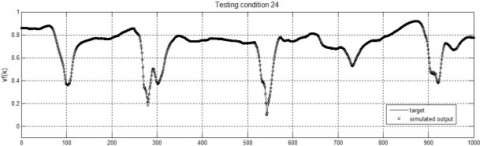## 3.2.png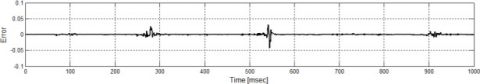## 3.3.png## 3.4.png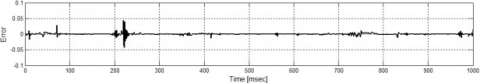Figure 3. Target vs simulated output and prediction error for: test 24 (churn flow) and test 27 (unstable slug flow)

Indeed, these represent high order and high frequency noisy components of the two-phase flow dynamics that cannot be predicted by the optimal NARMAX model in its present form. Parenthetically, this inability may possibly be determined by the choice of resampling the input-output considering one out of three time samples, which corresponds to cutting-off the high frequency disturbing dynamics.

The predictive ability shown and discussed with respect to results shown in Figure 1, 2 and 3 has been confirmed for the whole set of experimental conditions reported in Table 1. In order to verify the extent to which the neural model is able to predict the dynamics of the two-phase flow under study, the recursive prediction procedure proposed in  and  has been adopted. According to this procedure, the model prediction at a given time step k is fed back to the input layer, so as to be used in the input pattern of the neural network at time step k+1. Iterating q times such a feedback scheme corresponds to obtain a q-steps ahead prediction.

Figure 4 reports the comparisons between the target of the neural network and the predicted outputs obtained in the 3, 6 and 9 steps ahead predictions for the same experimental test discussed in Figure 1, which refer to the fundamental single- step ahead prediction. Parenthetically, recalling that the network input-output patterns were resampled with a sampling time step of 3 ms, the prediction horizons of results reported in Figure 4 are respectively of 9, 18 and 27 ms.

(a)

## 4.1.png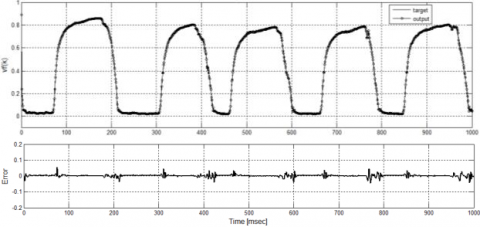(b)

## 4.2.png(c)

## 4.3.pngFigure 4. Experimental target vs simulated output for multiple prediction steps, q: (a) q=3; (b) q=6, (c) q=9

From the analysis of these plots it is possible to observe that, as expected, the predictive capabilities of the model progressively diminish with the increase of the prediction horizon. Nonetheless, by comparing the plots in Figure 1 (a) and (b) with the plots in Figure 4 (a), it emerges that for q=3 a very limited worsening of the prediction error occurs and, in fact, the model output satisfactory predicts the experimental target.

The results for q=6, reported in Figure 4 (b), show that, though affected by a greater error, the model is still able to predict the dominant patterns of the dynamics of the void fraction; therefore, a prediction horizon q=6 can still be appropriate for the design of a diagnostic system, especially if a lower accuracy can be admitted with respect to secondary noise-like fluid-dynamic phenomena, typically characterised by higher frequencies and lower amplitudes.

Finally, from the analysis of the results for q=9 reported in Figure 4 (c) it is apparent that the fitting between the desired target and the output of the model prediction is unsatisfactory and, therefore, the prediction error is high, showing that such a prediction horizon cannot be reached. It is worth to recall that this is not an inherent limitation of the proposed strategy but is a consequence of the intrinsic short-term predictability of the involved phenomena, which are strongly nonlinear.

Reported results show the potential of the proposed strategy for the assessment of a diagnostic tool for the monitoring of two-phase flows. With respect to the methodology in itself, it is worth observing that the same approach can be proposed:

– for other classes of two-phase flows, e.g., for gas-solid, liquid-solid or liquid-liquid flows;

– for pipes or, in a broader sense, plant configuration, characterised by generic inclination of the flow direction and, correspondingly, for a variety of possible flow patterns,

– for any choice of the monitoring variable chosen for the characterisation of two-phase flow dynamics and of the adopted measuring technique.

Moreover, predictive capabilities of the generalised NARMAX model can be configured within more sophisticated diagnostic tools, such as Control Charts algorithms aiming at the early detection of undesired or off- design operating conditions , or can be used to improve reliability and adaptability of performances of experiment- based analytical tools .

5. Conclusions

The aim of this study was to propose a novel tool for a flexible and computationally light modeling of the dynamics of two-phase flows, which are intrinsically complex due to the relevant number of degree of freedom and the nonlinear interaction of several phenomena. In order to assess such a tool, a generalized NARMAX model was implemented by means of artificial neural networks, which were trained and tested through a set of void fraction time series detected during an extensive experimental campaign for the characterization of vertical upward air-water two-phase flows under variable water and air superficial velocities, wsl and wsg. The proposed strategy is particularly appropriate for the description of nonlinear dynamics of unknown but even relatively high order; in fact, in the present study, in order to assess the optimal order, this was progressively increased until optimal prediction performances were observed for the model of the sixth order. For such a model, reported results show satisfactory agreement between the predicted and the experimental void fraction over the entire range of testing conditions, encompassing from the cap flow to the slug, plug and churn flow.

In order to verify the extent to which the prediction horizon of the model can be increased, the neural model was tested in a recursive scheme. This allowed to show that, though predictive capabilities are limited due to the intrinsic short- term predictability of complex dynamics, a horizon of up to six steps ahead was able to guaranteed satisfactory predictive performances. In particular, these were observed to provide a sufficiently accurate description of the dominant patterns of the experimental void fraction, with an error that is mainly due to an inadequate modelling of high order noisy components, corresponding to secondary fluidynamic patterns such as, for example, dispersed bubbles aerating the liquid phase. Hence, the generalized NARMAX strategy can be indeed implemented in advanced diagnostic tools for two- phase flows characterization. With this respect, the proposed approach can be flexibly modified in view of its application to other type of two-phase flows, independently on the differences concerning the nature of the two phases, the geometrical configuration of the plant, or the characteristics of the experimental time series chosen for monitoring the two-phase flow.

Nomenclature
 k Time sample nu Number of lagged inputs to the model ny Model order q Number of prediction steps u Input at the dynamical system vf Void fraction wsg Superficial gas velocity wsl Superficial liquid velocity y Output of the dynamical system Greek symbols e Prediction error fee Autocorrelation of the prediction error
References

 Li M., Liao R., Luo W., Dong, Y. (2016). Improved aziz prediction model of pressure gradient for multiphase flow in wells, International Journal of Heat and Technology, Vol. 34, No. 3, pp. 423-428.DOI: 10.18280/ijht.340

 Osman E.A., Aggour M.A. (2002). Artificial neural network model for accurate prediction of pressure drop in horizontal and near-horizontal-multiphase flow,Petroleum Science and Technology, Vol. 20, pp. 1–15.DOI: 10.1081/LFT-120002082

 Shippen M.E., Scott S.L. (2002). A neural network model for prediction of liquid holdup in two-phase horizontal flow, Proc. SPE Ann. Tech. Conf. Exh., San Antonio, Texas, Paper SPE-77499. DOI:10.2118/77499-MS

 Alizadehdakhel A., Rahimi M., Sanjari J., AlsairafiA.A. (2009). CFD and artificial neural network modeling of two-phase flow pressure drop,International Communication in Heat and MassTransfer, Vol. 36, pp. 850–856. DOI:10.1016/j.icheatmasstransfer.2009.05.005

 Castillo A.Á.D., Santoyo E., Garcia-Valladare O.(2012). A new void fraction correlation inferred from artificial neural networks for modeling two-phase flows in geothermal wells, Computers & Geosciences,Vol. 41, pp. 25–39. DOI:10.1016/j.cageo.2011.08.001

 Al-Wahaibi T., Mjalli F.S. (2014). Prediction of horizontal oil–water flow pressure gradient using artificial intelligence techniques, Chemical Engineering Communication, Vol. 201, pp. 209–224.DOI: 10.1080/00986445.2013.766603

 Azisi S., Awad M.M., Ahmadloo E. (2016). Prediction of water holdup in vertical and inclined oil–water twophase flow using artificial neural network,International Journal of Multiphase Flow, Vol. 80, pp.181–187. DOI:10.1016/j.ijmultiphaseflow.2015.12.010

 Sobhanifar N., Ahmadloo E., Azizi S. (2015).Prediction of two-phase heat transfer coefficients in a horizontal pipe for different inclined positions with artificial neural networks, ASME Journal of Heat Transfer, Vol. 137, Article No. (061009). DOI:10.1115/1.4029865

 Shirley R., Chakrabarti D.P., Das G. (2012). Artificial neural networks in liquid–liquid two-phase flow,Chemical Engineering Communication, Vol. 199, pp.1520–1542. DOI: 10.1080/00986445.2012.682323

 Xiea T., Ghiaasiaana S., Karrila S. (2004). Artificial neural network approach for flow regime classification in gas–liquid–fiber flows based on frequency domain analysis of pressure signals, Chemical Engineering Science, Vol. 59, No. 11, pp. 2241–2251. DOI:10.1016/j.ces.2004.02.017

 Rosa E., Salgado R., Ohishi T., Mastelari N. (2010).Performance comparison of artificial neural networks and expert systems applied to flow pattern identification in vertical ascendant gas–liquid flows,International Journal of Multiphase Flow, Vol. 36, No.9, pp. 738–754. DOI:10.1016/j.ijmultiphaseflow.2010.05.001

 El-Sebakhy E.A. (2010). Flow regimes identification and liquid-holdup prediction in horizontal multiphase flow based on neuro-fuzzy inference systems,Mathematics and Computers in Simulation, Vol. 80 No. 9, pp. 1854–1866. DOI:10.1016/j.matcom.2010.01.002

 Tenglong C., Guanghui S., Suizheng Q., Wenxi T. (2013). Applications of ANNs in flow and heat transfer problems in nuclear engineering: a review work, Progress in Nuclear Energy, Vol. 62, pp. 54-71.DOI: 10.1016/j.pnucene.2012.09.003

 Aarsnes U.J.F., Flatten T., Aamo O.M. (2016). Review of two-phase flow models for control and estimation,Annual Reviews in Control, Vol. 42, pp. 50–62. DOI:10.1016/j.arcontrol.2016.06.001

 Chen S., Billings S.A., Grant P.M. (1989).Representations of non-linear system: the NARMAX Model, International Journal of Control, Vol. 49, No.5, pp. 1013-1032.

 Narendra K.S., Parthasarathy K. (1990). Identification and control of dynamical systems using neural networks, IEEE Transactions on Neural Networks,Vol. 1, No. 1, pp. 4–21. DOI: 10.1109/72.80202

 Cantelli L., Fichera A., Pagano A. (2011). A highresolution resistive probe for nonlinear analysis of two-phase flows, Journal of Thermodynamics, Vol.2011, paper 491350, p. 10. DOI:10.1155/2011/491350

 Fichera A., Pagano A. (2015). Assessment of an identification strategy for the prediction of the dynamics of two-phase flows, Energy Procedia, Vol. 82, pp. 316-321. DOI: 10.1016/j.egypro.2015.12.039

 Leontaritis I.J., Billings S.A. (1985). Input-output parametric models for nonlinear systems. Part I: Deterministic non-linear systems, International Journal of Control, Vol. 41, No. 2, pp. 303-328. DOI:10.1080/0020718508961129

 Cammarata L., Fichera A., Pagano A. (2002). Neural prediction of combustion instability, Applied Energy,Vol. 72, pp. 513-528. DOI: 10.1016/S0306-2619(02)00024-7

 Fichera A., Pagano A. (2002). Neural network-based prediction of the oscillating behaviour of a closed loop241thermosiphon, International Journal of Heat and Mass Transfer, Vol. 45, pp. 3875-3884. DOI: 10.1016/S0017-9310(02)00095-9

 Cybenko G. (1989). Approximation by superpositionsof a sigmoidal function, Mathematics of Control Signals and Systems, Vol. 2, pp. 303-314. DOI:10.1007/BF02551274

 Funahashi K. (1989). On the approximate realization of continuous mapping by neural networks, Neural Networks, Vol. 2, pp. 183-192. DOI: 10.1016/0893-6080(89)90003-8

 Chen S., Billings S.A., Grant P.M. (1990). Non-linear system identification using neural networks,International Journal of Control, Vol. 51, No. 6, pp.1191-1214.

 Fichera A., Pagano A. (2013). A dynamics-based tool for the analysis of experimental two-phase flows,International Journal of Heat and Fluid Flow, Vol. 44,pp. 735-744.DOI: 10.1016/j.ijheatfluidflow.2013.10.003

 Boggs P.T., Byrd R.H., Schnabel R.B. (1987). Astable and efficient algorithm for nonlinear orthogonal distance regression, SIAM Journal on Scientific Statistical Computation, Vol. 8, pp. 1052–1078. DOI:10.1137/0908085

 Fichera A., Pagano A. (2009). Monitoring combustion unstable dynamics by means of control charts, Applied Energy, Vol. 86, No. 9, pp. 1574-1581. DOI: 10.1016/j.apenergy.2008.11.036

 Luo W., Li Y., Qinghua W., Li J., Liao R., Liu Z.(2016). Experimental study of gas-liquid two-phase flow for high velocity in inclined medium size tube and verification of pressure calculation methods,International Journal of Heat and Technology, Vol.34, No. 3, pp. 455-464. DOI: 10.18280/ijht.340315